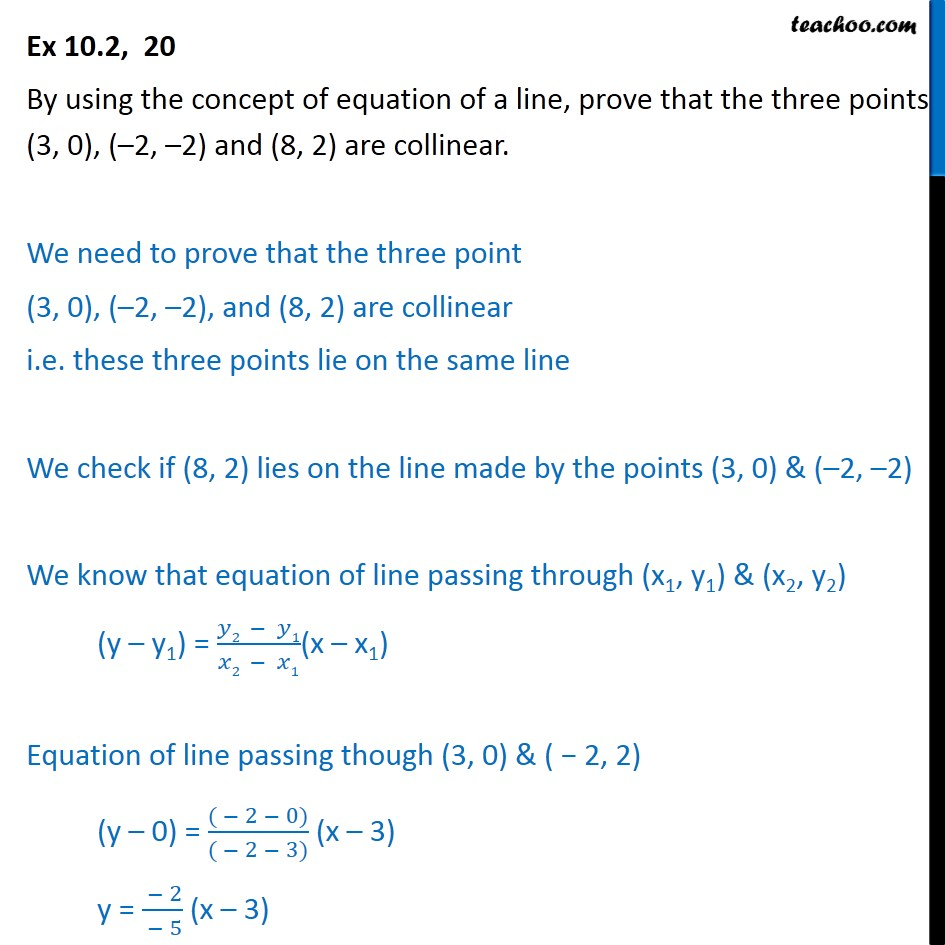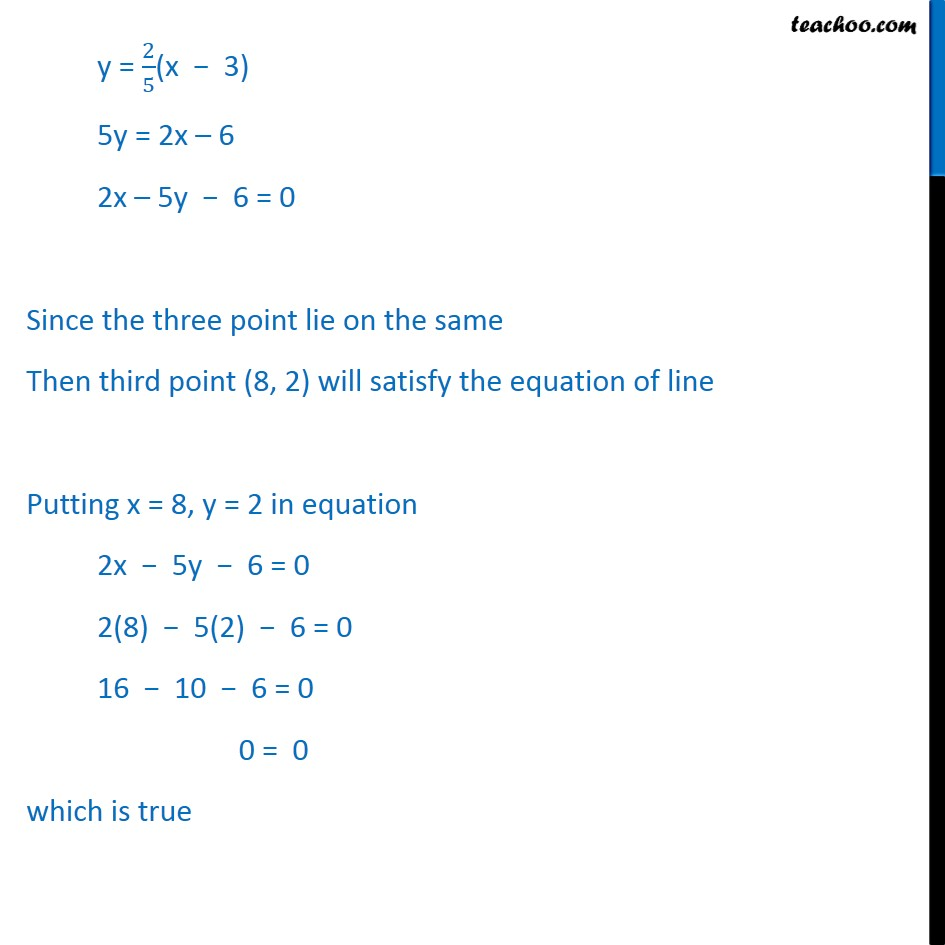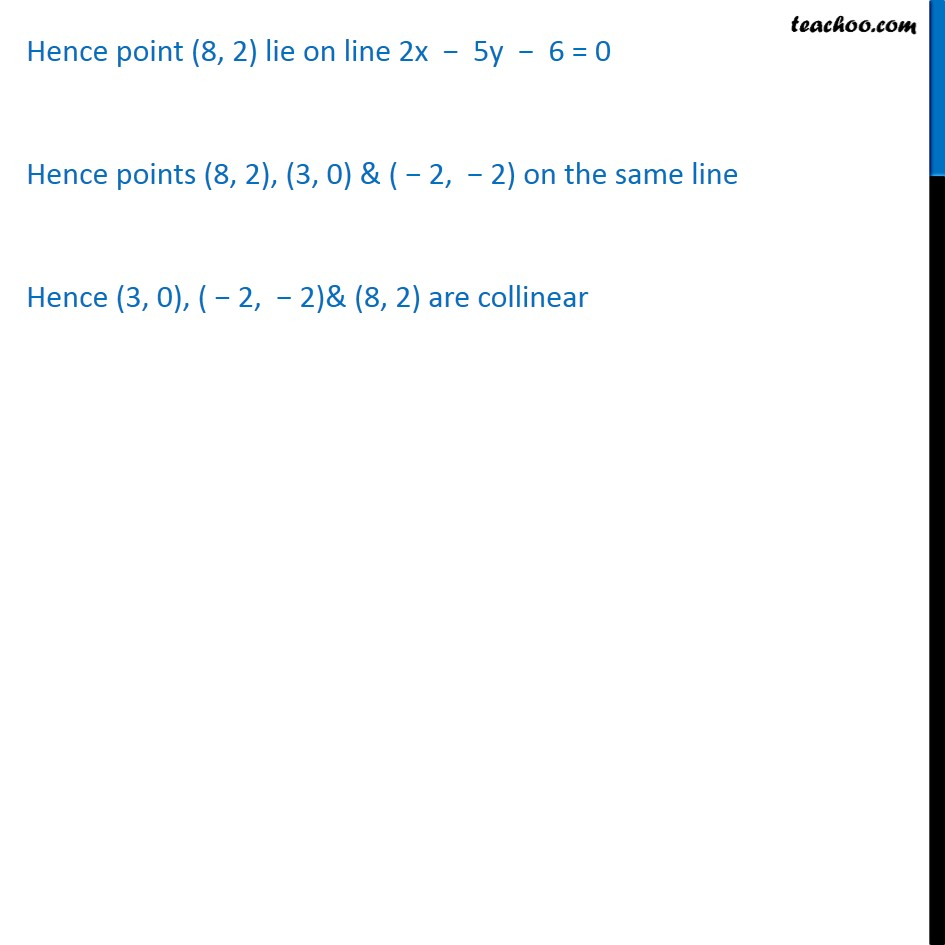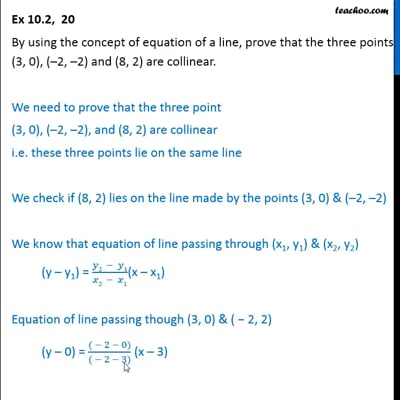Ex 10.2

Chapter 10 Class 11 Straight Lines
Serial order wiseThis video is only available for Teachoo black users

Maths Crash Course - Live lectures + all videos + Real time Doubt solving!

### Transcript

Ex 10.2, 20 By using the concept of equation of a line, prove that the three points (3, 0), ( 2, 2) and (8, 2) are collinear. We need to prove that the three point (3, 0), ( 2, 2), and (8, 2) are collinear i.e. these three points lie on the same line We check if (8, 2) lies on the line made by the points (3, 0) & ( 2, 2) We know that equation of line passing through (x1, y1) & (x2, y2) (y y1) = 2 1 2 1 (x x1) Equation of line passing though (3, 0) & ( 2, 2) (y 0) = ( 2 0) ( 2 3) (x 3) y = 2 5 (x 3) y = 2 5 (x 3) 5y = 2x 6 2x 5y 6 = 0 Since the three point lie on the same Then third point (8, 2) will satisfy the equation of line Putting x = 8, y = 2 in equation 2x 5y 6 = 0 2(8) 5(2) 6 = 0 16 10 6 = 0 0 = 0 which is true Hence point (8, 2) lie on line 2x 5y 6 = 0 Hence points (8, 2), (3, 0) & ( 2, 2) on the same line Hence (3, 0), ( 2, 2)& (8, 2) are collinear